## Selina Concise Mathematics Class 7 ICSE Solutions Chapter 13 Set Concepts (Some Simple Divisions by Vedic Method)

Selina Publishers Concise Mathematics Class 7 ICSE Solutions Chapter 13 Set Concepts (Some Simple Divisions by Vedic Method)

### Set Concepts Exercise 13A – Selina Concise Mathematics Class 7 ICSE Solutions

Question 1.
Find, whether or not, each of the following collections represent a set:
(i) The collection of good students in your school.
(ii) The collection of the numbers between 30 and 45.
(iii) The collection of fat-people in your colony.
(iv) The collection of interesting books in your school library.
(v) The collection of books in the library and are of your interest.
Solution:Question 2.
State whether true or false :
(i) Set {4, 5, 8} is same as the set {5, 4, 8} and the set {8, 4, 5}
(ii) Sets {a, b, m, n} and {a, a, m, b, n, n) are same.
(iii) Set of letters in the word ‘suchismita’ is {s, u, c, h, i, m, t, a}
(iv) Set of letters in the word ‘MAHMOOD’ is {M, A, H, O, D}.
Solution:Question 3.
Let set A = {6, 8, 10, 12} and set B = {3, 9, 15, 18}.
Insert the symbol ‘ ∈ ’ or ‘ ∉ ’ to make each of the following true :
(i) 6 …. A
(ii) 10 …. B
(iii) 18 …. B
(iv) (6 + 3) …. B
(v) (15 – 9) …. B
(vi) 12 …. A
(vii) (6 + 8) …. A
(viii) 6 and 8 …. A
Solution:Question 4.
Express each of the following sets in
roster form :
(i) Set of odd whole numbers between 15 and 27.
(ii) A = Set of letters in the word “CHITAMBARAM”
(iii) B = {All even numbers from 15 to 26}
(iv) P = {x : x is a vowel used in the word ‘ARITHMETIC’}
(v) S = {Squares of first eight whole numbers}
(vi) Set of all integers between 7 and 94; which are divisible by 6.
(vii) C = {All composite numbers between 2 and 20}
(viii) D = Set of Prime numbers from 2 to 23.
(ix) E = Set of natural numbers below 30 which are divisible by 2 or 5.
(x) F = Set of factors of 24.
(xi) G = Set of names of three closed figures in Geometry.
(xii) H = {x : x eW and x < 10}
(xiii) J = {x: x e N and 2x – 3 ≤17}
(xiv) K = {x : x is an integer and – 3 < x < 5}
Solution: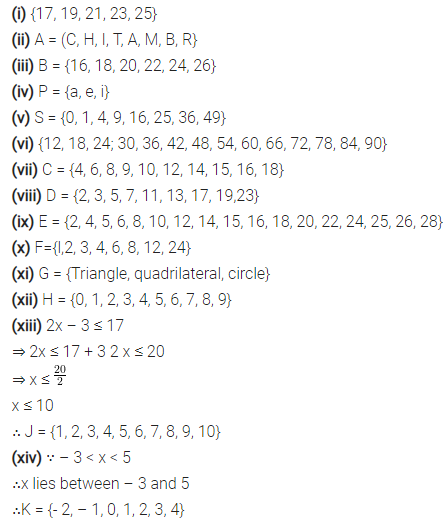Question 5.
Express each of the following sets in set- builder notation (form) :
(i) {3, 6, 9, 12, 15}
(ii) {2, 3, 5, 7, 11, 13 …. }
(iii) {1, 4, 9, 16, 25, 36}
(iv) {0, 2, 4, 6, 8, 10, 12, …. }
(v) {Monday, Tuesday, Wednesday}
(vi) {23, 25, 27, 29, … }
(vii) {$$\frac { 1 }{ 3 }$$,$$\frac { 1 }{ 4 }$$,$$\frac { 1 }{ 5 }$$,$$\frac { 1 }{ 6 }$$,$$\frac { 1 }{ 7 }$$,$$\frac { 1 }{ 8 }$$}
(viii) {42, 49, 56, 63, 70, 77}
Solution: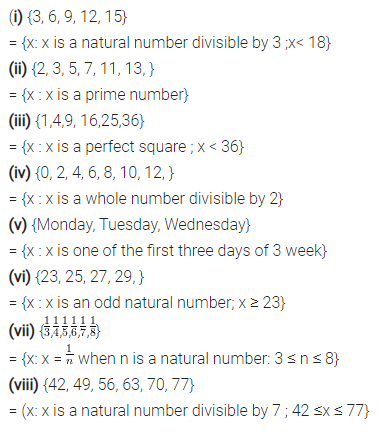Question 6.
Given : A = {x : x is a multiple of 2 and is less than 25}
B = {x : x is a square of a natural number and is less than 25}
C = {x : x is a multiple of 3 and is less than 25}
D = {x: x is a prime number less than 25}
Write the sets A, B, C and D in roster form.
Solution: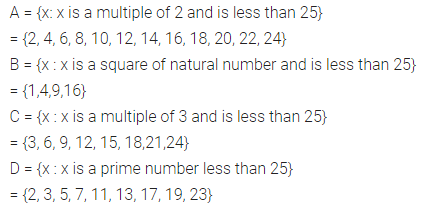### Set Concepts Exercise 13B – Selina Concise Mathematics Class 7 ICSE Solutions

Question 1.
Write the cardinal number of each of the following sets:
(i) A = Set of days in a leap year.
(ii) B = Set of numbers on a clopk-face.
(iii) C = {x : x ∈ N and x ≤ 7}
(iv) D = Set of letters in the word “PANIPAT”.
(v) E = Set of prime numbers between 5 and 15.
(vi) F = {x : x ∈ Z and – 2 < x ≤ 5}
(vii) G = {x : x is a perfect square number, x ∈N and x ≤ 30}.
Solution:Question 2.
For each set, given below, state whether it is finite set, infinite set or the null set :
(i) {natural numbers more than 100}
(ii) A = {x : x is an integer between 1 and 2}
(iii) B = {x : x ∈ W ; x is less than 100}.
(iv) Set of mountains in the world.
(v) {multiples of 8}.
(vi) {even numbers not divisible by 2}.
(vii) {squares of natural numbers}.
(viii) {coins used in India}
(ix) C = {x | x is a prime number between 7 and 10}.
(x) Planets of the Solar system.
Solution:Question 3.
State, which of the following pairs of sets are disjoint :
(i) {0, 1, 2, 6, 8} and {odd numbers less than 10.
(ii) {birds} and {tress}
(iii) {x : x is a fan of cricket} and {x : x is a fan of football}.
(iv) A = {natural numbers less than 10} and B = {x : x is a multiple of 5}.
(v) {people living in Calcutta} and {people living in West Bengal}.
Solution:Question 4.
State whether the given pairs of sets are equal or equivalent.
(i) A = {first four natural numbers} and B = {first four whole numbers}.
(ii) A = Set of letters of the word “FOLLOW” and B = Set of letters of the word “WOLF”.
(iii) E = {even natural numbers less than 10} and O = {odd natural numbers less than 9}
(iv) A = {days of the week starting with letter S} and B = {days of the week starting with letter T}.
(v) M = {multiples of 2 and 3 between 10 and 20} and N = {multiples of 2 and 5 between 10 and 20}.
(vi) P = {prime numbers which divide 70 exactly} and Q = {prime numbers which divide 105 exactly}
(vii) A = {0², 1², 2², 3², 4²} and = {16, 9,4, 1, 0}.
(viii) E = {8,JO, 12, 14, 16} and F = {even natural numbers between 6 and 18}.
(ix) A = {letters of the word SUPERSTITION} and B = {letters of the word JURISDICTION}.
Solution: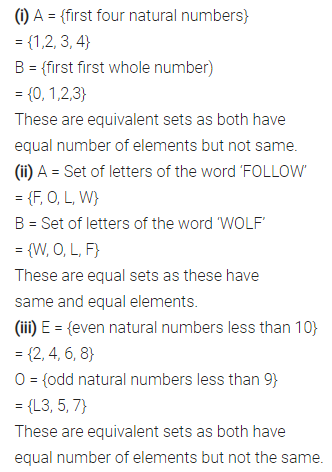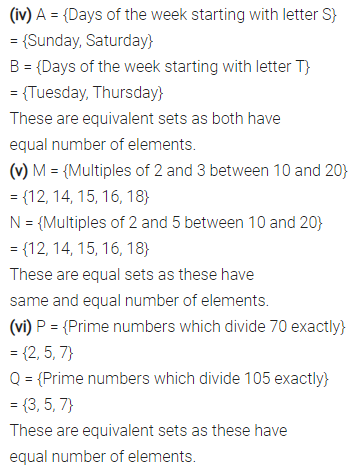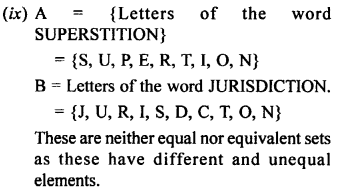Question 5.
Examine which of the following sets are the empty sets :
(i) The set of triangles having three equal sides.
(ii) The set of lions in your class.
(iii) {x : x + 3 = 2 and xeN}
(iv) P = {x : 3x = 0}
Solution:Question 6.
State true or false :
(i) All examples of the empty set are equal.
(ii) All examples of the empty set are equivalent.
(iii) If two sets have the same cardinal number, they are equal sets.
(iv) If n (A) = n (B) then A and B are equivalent sets.
(v) If B = {x : x + 4 = 4}, then B is the empty set.
(vi) The set of all points in a line is a finite set.
(vii) The set of letters in your Mathematics book is an infinite set.
(viii) If M = {1, 2, 4, 6} and N = {x : x is a factor of 12} ; then M = N.
(ix) The set of whole numbers greater than 50 is an infinite set.
(x) If A and B are two different infinite sets, then n (A) = n (B).
Solution: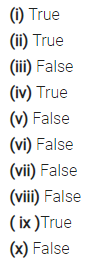Question 7.
Which of the following represent the null set ?
φ, {0}, 0, { }, {φ}
Solution: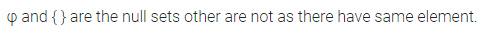### Set Concepts Exercise 13C – Selina Concise Mathematics Class 7 ICSE Solutions

Question 1.
Fill in the blanks :
(i) If each element of set P is also an element of set Q, then P is said to be …… of Q and Q is said to be of P.
(ii) Every set is a ….. of itself.
(iii) The empty set is a …… of every set.
(iv) If A is proper subset of B, then n (A) …. n (B).
Solution: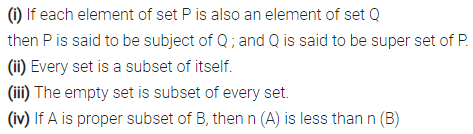Question 2.
If A = {5, 7, 8, 9} ; then which of the following are subsets of A ?
(i) B = {5, 8}
(ii) C = {0}
(iii) D = {7, 9, 10}
(iv) E = { }
(V) F = {8, 7, 9, 5}
Solution: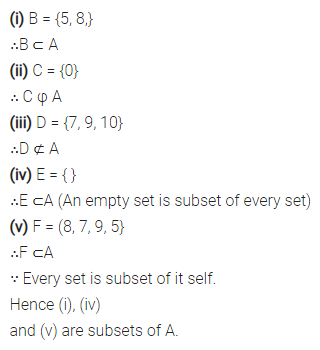Question 3.
If P = {2, 3, 4, 5} ; then which of the following are proper subsets of P ?
(i) A = {3, 4}
(ii) B = { }
(iii) C = {23, 45}
(iv) D = {6, 5, 4}
(v) E = {0}
Solution: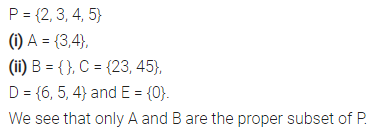Question 4.
If A = {even numbers less than 12},
B = {2, 4},
C = {1, 2, 3},
D = {2, 6} and E = {4}
State which of the following statements are true :
(i) B⊂A
(ii) C⊆A
(iii) D⊂C
(iv) D ⊄ A
(v)E⊇B
(vi) A⊇B⊇E
Solution: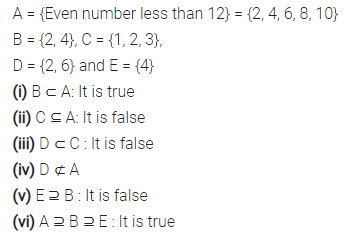Question 5.
Given A = {a, c}, B = {p, q, r} and C = Set of digits used to form number 1351.
Write all the subsets of sets A, B and C.
Solution:Question 6.
(i) If A = {p, q, r}, then number of subsets of A = ……
(ii) If B = {5, 4, 6, 8}, then number of proper subsets of B = ……
(iii) If C = {0}, then number of subsets of C = …..
(iv) If M = {x : x ∈ N and x < 3}, then M has …… proper subsets.
Solution:Question 7.
For the universal set {4, 5, 6, 7, 8, 9, 10, 11,12,13} ; find its subsets A, B, C and D such that
(i) A = {even numbers}
(ii) B = {odd numbers greater than 8}
(iii) C = {prime numbers}
(iv) D = {even numbers less than 10}.
Also, find compliments of each set i.e., find A’, B’, C’ and D’.
Solution: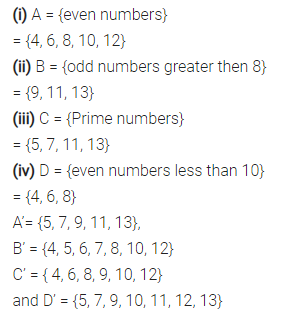### Set Concepts Exercise 13D – Selina Concise Mathematics Class 7 ICSE Solutions

Question 1.
If A = {4, 5, 6, 7, 8} and B = {6, 8, 10, 12}, find :
(i) A∪B
(ii) A∩B
(iii) A-B
(iv) B-A
Solution: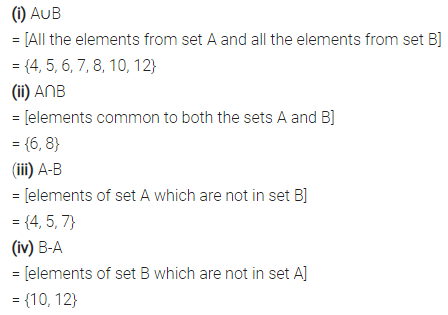Question 2.
If A = {3, 5, 7, 9, 11} and B = {4, 7, 10}, find:
(i) n(A)
(ii) n(B)
(iii) A∪B and n(A∪B)
(iv) A∩B and n(A∩B)
Solution:Question 3.
If A = {2, 4, 6, 8} and B = {3, 6, 9, 12}, find:
(i) (A ∩ B) and n(A ∩ B)
(ii) (A – B) and n(A – B)
(iii) n(B)
Solution: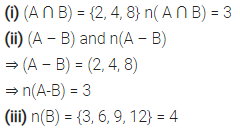Question 4.
If P = {x : x is a factor of 12} and Q = {x: x is a factor of 16}, find :
(i) n(P)
(ii) n(Q)
(iii) Q – P and n(Q – P)
Solution: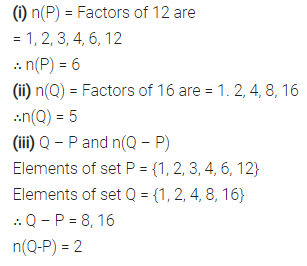Question 5.
M = {x : x is a natural number between 0 and 8) and N = {x : x is a natural number from 5 to 10}. Find :
(i) M – N and n(M – N)
(ii) N – M and n(N – M)
Solution: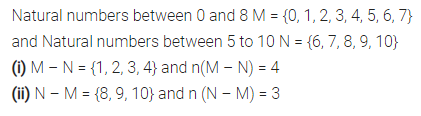Question 6.
If A = {x: x is natural number divisible by 2 and x< 16} and B = {x:x is a whole number divisible by 3 and x < 18}, find :
(i) n(A)
(ii) n(B)
(iii) A∩B and n(A∩B)
(iv) n(A – B)
Solution: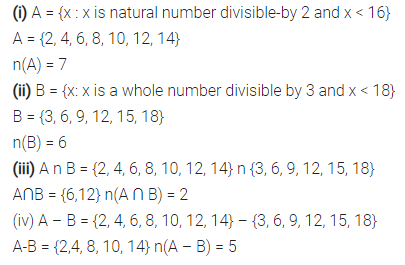Question 7.
Let A and B be two sets such that n(A) = 75, M(B) = 65 and n(A ∩ B) = 45, find :
(i) n(A∪ B)
(ii) n(A – B)
(iii) n(B – A)
Solution: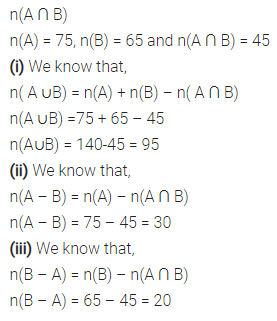Question 8.
Let A and B be two sets such that n(A) = 45, n(B) = 38 and n(A ∪B) = 70, find :
(i) n(A∩B)
(ii) n(A-B)
(iii) n(B – A)
Solution:Question 9.
Let n(A) 30, n(B) = 27 and n(A∪B) = 45, find :
(i) n(A∩B)
(ii) n(A-B)
Solution: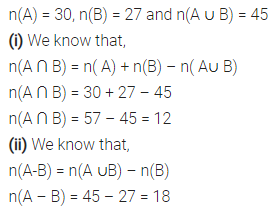Question 10.
Let n(A) = 31, n(B) = 20 and n(A ∩ B) = 6, find:
(i) n(A-B)
(ii) n(B – A)
(iii) n(A ∪B)
Solution: